# GSEB Solutions Class 6 Maths Chapter 7 Fractions Ex 7.5

Gujarat Board GSEB Textbook Solutions Class 6 Maths Chapter 7 Fractions Ex 7.5 Textbook Questions and Answers.

## Gujarat Board Textbook Solutions Class 6 Maths Chapter 7 Fractions Ex 7.5

Question 1.
Write these fractions appropriately as additions or subtractions:Solution:
(a) The given figure represents an addition of $$\frac { 1 }{ 5 }$$ and $$\frac { 2 }{ 5 }$$ pictorially, i.e.
$$\frac { 1 }{ 5 }$$ + $$\frac { 2 }{ 5 }$$ = $$\frac { 3 }{ 5 }$$
The given figure will be as under:(b) Here, the given figure represents a subtraction $$\frac { 3 }{ 5 }$$ of from 1, i.e.
1 – $$\frac { 3 }{ 5 }$$ = $$\frac { 2 }{ 5 }$$
The given figure will be as under: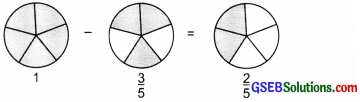(e) The given figure represents addition of $$\frac { 2 }{ 6 }$$ and $$\frac { 3 }{ 6 }$$, i.e $$\frac { 2 }{ 6 }$$ + $$\frac { 3 }{ 6 }$$ = $$\frac { 5 }{ 6 }$$
The given figure is represented as under: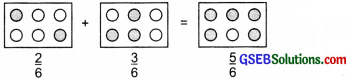Question 2.
Solve: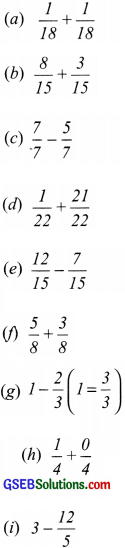Solution:(g) Since 1 and $$\frac { 3 }{ 3 }$$ are equivalent fractions.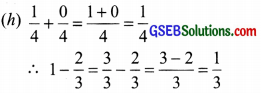(i) Since 3 = $$\frac { 3 }{ 1 }$$ and equivalent fraction of $$\frac { 3 }{ 1 }$$ having denominator as 5 is given by
$$\frac { 3 }{ 1 }$$ x $$\frac { 5 }{ 5 }$$ = $$\frac { 15 }{ 5 }$$
3 – $$\frac { 12 }{ 5 }$$ = $$\frac { 15 }{ 5 }$$ – $$\frac { 12 }{ 5 }$$ = $$\frac { 15-12 }{ 5 }$$ = $$\frac { 3 }{ 5 }$$Question 3.
Shuhham painted $$\frac { 2 }{ 3 }$$ of the wall space in his room. His sister Macihavi helped and painted $$\frac { 1 }{ 3 }$$ of the wail space. How much did they paint together ?
Solution:
Portion of the wall painted by Shubham = $$\frac { 2 }{ 3 }$$
Portion of the wall painted by Madhavi = $$\frac { 1 }{ 3 }$$
The portion of wall painted = $$\frac { 2 }{ 3 }$$ + $$\frac { 1 }{ 3 }$$ = $$\frac { 2+1 }{ 3 }$$ = $$\frac { 3 }{ 3 }$$ or 1
Thus, Shubham and Madhavi together painted the complete wall.

Question 4.
Fill in the missing fractions.Solution: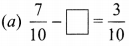The ‘missing fraction’ is less than $$\frac { 7 }{ 10 }$$ by $$\frac { 3 }{ 10 }$$
The ‘missing fraction’ = $$\frac { 7 }{ 10 }$$ – $$\frac { 3 }{ 10 }$$
= $$\frac { 7-3 }{ 10 }$$ = $$\frac { 4 }{ 10 }$$ or $$\frac { 2 }{ 5 }$$
We have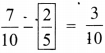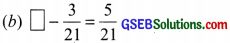The ‘missing fractions is more than $$\frac { 3 }{ 21 }$$ by $$\frac { 5 }{ 21 }$$
The sum of $$\frac { 3 }{ 21 }$$ and $$\frac { 5 }{ 21 }$$ must be equal to the missing fraction.
Missing fraction = $$\frac { 3 }{ 21 }$$ + $$\frac { 5 }{ 21 }$$ = $$\frac { 3+5 }{ 21 }$$ = $$\frac { 8 }{ 21 }$$
We have: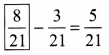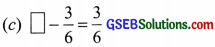The ‘missing fraction’ is more than $$\frac { 3 }{ 6 }$$ by $$\frac { 3 }{ 6 }$$
Missing fraction $$\frac { 3 }{ 6 }$$ + $$\frac { 3 }{ 6 }$$ = $$\frac { 3+3 }{ 6 }$$ = $$\frac { 6 }{ 6 }$$ = 1
We have:Since, the sum of the ‘missing fraction’ and $$\frac { 5 }{ 27 }$$ is $$\frac { 12 }{ 27 }$$.
The missing fraction = $$\frac { 12 }{ 27 }$$ – $$\frac { 5 }{ 27 }$$ = $$\frac { 12-5 }{ 27 }$$ = $$\frac { 7 }{ 27 }$$
Thus,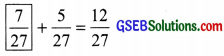Question 5.
Javed was given $$\frac { 5 }{ 7 }$$ of a basket of oranges. What fraction of oranges was left in the basket?
Solution:
Let the basket full of oranges be denoted by 1.
Portion of oranges given to Javed = $$\frac { 5 }{ 7 }$$
Portion of oranges left in the basket 1 – $$\frac { 5 }{ 7 }$$ = $$\frac { 7 }{ 7 }$$ – $$\frac { 5 }{ 7 }$$ = $$\frac { 7-5 }{ 7 }$$ = $$\frac { 2 }{ 7 }$$
[1and $$\frac { 7 }{ 7 }$$ are equivalent fractions]
$$\frac { 2 }{ 7 }$$ of oranges was left in the basket.